### Visualizing 3-d In 2-d Class 8th Mathematics AP Board Solution

##### Question 1.Count the number of faces , vertices , and edges of given polyhedra and verify Euler’s formula.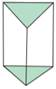Answer:Here,No. of Faces (F):5No. of Vertices (V):6No. of Edges (E):9Euler’s formula: F + V = E + 2⇒ 5 + 6 = 9 + 2⇒ 11 = 11∴ Euler’s formula satisfies for the shape.Question 2.Count the number of faces , vertices , and edges of given polyhedra and verify Euler’s formula.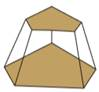Answer:Here,No. of Faces (F):7No. of Vertices (V):10No. of Edges (E):15Euler’s formula: F + V = E + 2⇒ 7 + 10 = 15 + 2⇒ 17 = 17∴ Euler’s formula satisfies for the shape.Question 3.Count the number of faces , vertices , and edges of given polyhedra and verify Euler’s formula.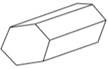Answer:Here,No. of Faces (F):8No. of Vertices (V):12No. of Edges (E):18Euler’s formula: F + V = E + 2⇒ 8 + 12 = 18 + 2⇒ 20 = 20∴ Euler’s formula satisfies for the shape.Question 4.Count the number of faces , vertices , and edges of given polyhedra and verify Euler’s formula.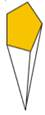Answer:Here,No. of Faces (F):6No. of Vertices (V):6No. of Edges (E):10Euler’s formula: F + V = E + 2⇒ 6 + 6 = 10 + 2⇒ 12 = 12∴ Euler’s formula satisfies for the shape.Question 5.Count the number of faces , vertices , and edges of given polyhedra and verify Euler’s formula.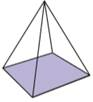Answer:Here,No. of Faces (F):5No. of Vertices (V):5No. of Edges (E):8Euler’s formula: F + V = E + 2⇒ 5 + 5 = 8 + 2⇒ 10 = 10∴ Euler’s formula satisfies for the shape.Question 6.Count the number of faces , vertices , and edges of given polyhedra and verify Euler’s formula.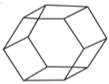Answer:Here,No. of Faces (F):8No. of Vertices (V):12No. of Edges (E):18Euler’s formula: F + V = E + 2⇒ 8 + 12 = 18 + 2⇒ 20 = 20∴ Euler’s formula satisfies for the shape.Question 7.Count the number of faces , vertices , and edges of given polyhedra and verify Euler’s formula.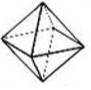Answer:Here,No. of Faces (F):8No. of Vertices (V):6No. of Edges (E):12Euler’s formula: F + V = E + 2⇒ 8 + 6 = 12 + 2⇒ 14 = 14∴ Euler’s formula satisfies for the shape.Question 8.Count the number of faces , vertices , and edges of given polyhedra and verify Euler’s formula.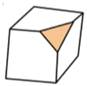Answer:Here,No. of Faces (F):7No. of Vertices (V):10No. of Edges (E):15Euler’s formula: F + V = E + 2⇒ 7 + 10 = 15 + 2⇒ 17 = 17∴ Euler’s formula satisfies for the shape.Question 9.Is a square prism and cube are same? explain.Answer:No, Every Square prism is not a Cube (∵ All faces in a square prism need not be identical), but conversely all cubes are Square prisms.Question 10.Can a polyhedra have 3 triangular faces only? explain.Answer:No, a polyhedra with 3 triangular faces does not exist,∵For a polyhedra with 3 triangular faces, there exist another triangular face.Question 11.Can a polyhedra have 4 triangular faces only? explain.Answer:Yes, a polyhedra with exactly 4 triangular faces can exist,A polyhedra with 4 triangular faces is triangular Pyramid.Question 12.Complete the table by using Euler’s formula.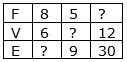Answer:(i) Given: Faces = 8Vertices = 6Edges = ?According to Euler’s formula, we know that : F + V = E + 2∴ 8 + 6 = E + 2⇒ 14 = E + 2⇒ E = 14—2 = 12∴ No. of Edges = 12(ii) Given: Faces = 5Vertices = ?Edges = 9According to Euler’s formula, we know that : F + V = E + 2∴ 5 + V = 9 + 2⇒ 5 + V = 11⇒ V = 11—5 = 6∴ No. of Vertices = 6(iii) Given: Faces = ?Vertices = 12Edges = 30According to Euler’s formula, we know that : F + V = E + 2∴ F + 12 = 30 + 2⇒ F + 12 = 32⇒ E = 32—12 = 20∴ No. of faces = 20Question 13.Can a polyhedra have 10 faces, 20 edges and 15 vertices?Answer:Given: No. of face(F):10No. of edges(E):20No. of vertices(V):15According to Euler formula, we know thatF + V = E + 2That is, here10 + 15 = 20 + 2⇒ 25 = 22This is not true.(That is, 25≠22 )∴ A polyhedra with 10 faces, 20 edges and 15 vertices is not possible.Question 14.Complete the following table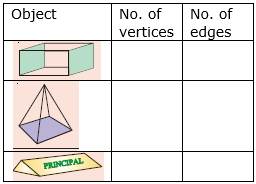Answer:1) A cuboid has 8 vertices and 12 edges.2) A square pyramid has 5 vertices and 8 edges.3) A triangular prism has 6 vertices and 9 edges.∴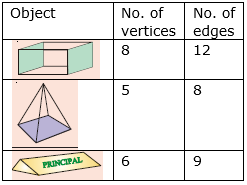Question 15.Name the 3-D objects or shapes that can be formed from the following nets.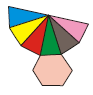Answer:No. of faces in the net: 7 (1 hexagon + 6 triangles)No. of vertices in the net: 7No. of edges in the net: 12∴The possible shape we get from the net is hexagonal pyramid.Question 16.Name the 3-D objects or shapes that can be formed from the following nets.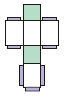Answer:No. of faces in the net: 6 (2 squares + 4 rectangles)No. of vertices in the net: 8No. of edges in the net: 12∴The possible shape we get from the net is Cuboid.Question 17.Name the 3-D objects or shapes that can be formed from the following nets.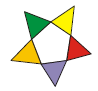Answer:No. of faces in the net:6(1 pentagon + 5 triangles)No. of vertices in the net: 6No. of edges in the net: 10∴The possible shape we get from the net is pentagonal pyramid.Question 18.Name the 3-D objects or shapes that can be formed from the following nets.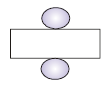Answer:No. of faces in the net: 3 (2 circles + 1 rectangle)No. of vertices in the net: 0No. of edges in the net: 2∴The possible shape we get from the net is Cylinder.Question 19.Name the 3-D objects or shapes that can be formed from the following nets.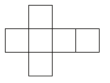Answer:No. of faces in the net: 6(6 squares)No. of vertices in the net: 8No. of edges in the net: 12∴The possible shape we get from the net Cube.Question 20.Name the 3-D objects or shapes that can be formed from the following nets.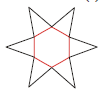Answer:No. of faces in the net:6(1 hexagon + 6 triangles)No. of vertices in the net: 7No. of edges in the net: 12∴The possible shape we get from the net is hexagonal pyramid.Question 21.Name the 3-D objects or shapes that can be formed from the following nets.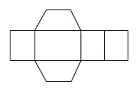Answer:No. of faces in the net:6(2 trapezium + 4 rectangles)No. of vertices in the net: 8No. of edges in the net: 12∴The possible shape we get from the net is trapezoid.Question 22.Draw the following diagram on the check ruled book and find out which of the following diagrams makes cube?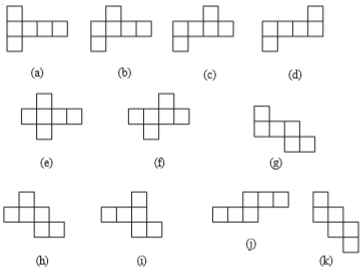Answer:(a)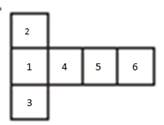i. Fold Square-2, 90° downwardsii. Fold Square-3, 90° downwardsiii. Fold Square-4, 90° downwardsiv. Now, fold Square-5, 90° left upwardv. Now, fold Square-6, 90° upwardHence, by the above procedure, we can form a cube with the given net.(b)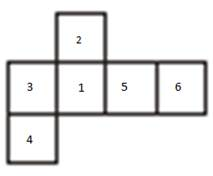i. Fold Square-2, 90° downwardsii. Fold Square-3, 90° downwardsiii. Fold Square-4, 90° rightiv. Now, fold Square-5, 90° downwardv. Now, fold Square-6, 90° left upwardHence, by the above procedure, we can form a cube with the given net.(c)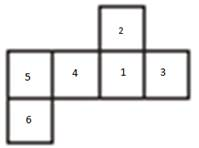i. Fold Square-2, 90° downwardsii. Fold Square-3, 90° downwardsiii. Fold Square-4, 90° downwardsiv. Now, fold Square-5, 90° right upwardv. Now, fold Square-6, 90° rightHence, by the above procedure we can form a cube with the given net.(d)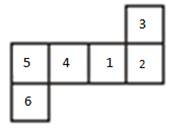i. Fold Square-2, 90° downwardsii. Fold Square-3, 90° leftiii. Fold Square-4, 90° downwardsiv. Now, fold Square-5, 90° right upwardv. Now, fold Square-6, 90° rightHence, by the above procedure we can form a cube with the given net.(e)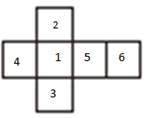i. Fold Square-2, 90° downwardsii. Fold Square-3, 90° downwardsiii. Fold Square-4, 90° downwardsiv. Fold Square-5, 90° downwardv. Now, fold Square-6, 90° left upwardHence, by the above procedure we can form a cube with the given net.(f)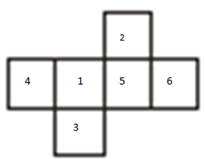i. Fold Square-3, 90° downwardsii. Fold Square-4, 90° downwardsiii. Fold Square-5, 90° downwardsiv. Now, fold Square-2 90° leftv. Now, fold Square-6 90° left upwardHence, by the above procedure we can form a cube with the given net.(g)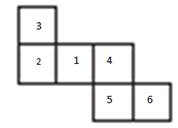i. Fold Square-2, 90° downwardsii. Fold Square-3, 90° rightiii. Fold Square-4, 90° downwardsiv. Now, fold Square-5 90° leftv. Now, fold Square-6 90° straight upwardHence, by the above procedure we can form a cube with the given net.(h)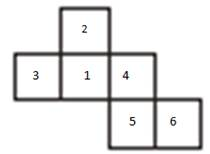i. Fold Square-2, 90° downwardsii. Fold Square-3, 90° downwardsiii. Fold Square-4, 90° downwardsiv. Now, fold Square-5 90° leftv. Now, fold Square-6 90° straight upwardHence, by the above procedure we can form a cube with the given net.(i)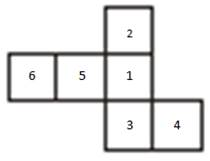i. Fold Square-2, 90° downwardsii. Fold Square-3, 90° downwardsiii. Fold Square-4, 90° left of square-3iv. Now, fold Square-5 90° downwardsv. Now, fold Square-6 90° right upwardHence, by the above procedure we can form a cube with the given net.(j)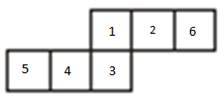i. Fold Square-2, 90° downwardsii. Fold Square-3, 90° downwardsiii. Fold Square-4, 90° right to square-3iv. Now, fold Square-5 90° right to square-4v. Now, fold Square-6 90° left upwardHence, by the above procedure we can form a cube with the given net.(k)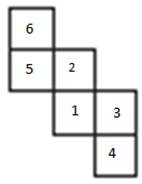i. Fold Square-6, 90° downwardsii. Fold Square-5, 90° downwardsiii. Fold Square-4, 90° downwardsiv. Now, fold Square-3 90° downwardsv. Now, fold Square-1 90° downwardsHence, by the above procedure we can form a cube with the given net.Question 23.Answer the following questions.(a) Name the polyhedron which has four vertices, four faces?(b) Name the solid object which has no vertex?(c) Name the polyhedron which has 12 edges?(d) Name the solid object which has one surface?(e) How is a cube different from cuboid?(f) Which two shapes have the same number of edges, vertices, and faces?(g) Name the polyhedron which has 5 vertices and 5 faces?Answer:(a) A polyhedron with 4 vertices and 4 faces is tetrahedron.(b) The only Solid object with no vertex is Sphere.(c) The possible polyhedrons with 12 edges are a cube, cuboid, Hexagonal Pyramid.(d) The Only solid object with 1 face is Sphere.(e) Cube is a Regular Polyhedron (All faces are identical)Whereas Cuboid is not a Regular Polyhedron (All faces are not identical)(f) Cube and Cuboid have a same number of faces, edges, vertices.That is 6 faces, 12 edges, 8 vertices.(g) A Polyhedron with 5 vertices and 5 faces is square Pyramid.Question 24.Write the names of the objects given below.(a)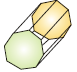(b)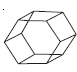(c)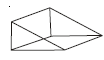(d)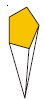Answer:(a) The given Object is Octagonal prism.(b) The given Object is Hexagonal prism.(c) The given Object is triangular prism.(d) The given Object is Pentagonal Pyramid.

PDF FILE TO YOUR EMAIL IMMEDIATELY PURCHASE NOTES & PAPER SOLUTION. @ Rs. 50/- each (GST extra)

HINDI ENTIRE PAPER SOLUTION

MARATHI PAPER SOLUTION

SSC MATHS I PAPER SOLUTION

SSC MATHS II PAPER SOLUTION

SSC SCIENCE I PAPER SOLUTION

SSC SCIENCE II PAPER SOLUTION

SSC ENGLISH PAPER SOLUTION

SSC & HSC ENGLISH WRITING SKILL

HSC ACCOUNTS NOTES

HSC OCM NOTES

HSC ECONOMICS NOTES

HSC SECRETARIAL PRACTICE NOTES

# 2019 Board Paper Solution

HSC ENGLISH SET A 2019 21st February, 2019

HSC ENGLISH SET B 2019 21st February, 2019

HSC ENGLISH SET C 2019 21st February, 2019

HSC ENGLISH SET D 2019 21st February, 2019

SECRETARIAL PRACTICE (S.P) 2019 25th February, 2019

HSC XII PHYSICS 2019 25th February, 2019

CHEMISTRY XII HSC SOLUTION 27th, February, 2019

OCM PAPER SOLUTION 2019 27th, February, 2019

HSC MATHS PAPER SOLUTION COMMERCE, 2nd March, 2019

HSC MATHS PAPER SOLUTION SCIENCE 2nd, March, 2019

SSC ENGLISH STD 10 5TH MARCH, 2019.

HSC XII ACCOUNTS 2019 6th March, 2019

HSC XII BIOLOGY 2019 6TH March, 2019

HSC XII ECONOMICS 9Th March 2019

SSC Maths I March 2019 Solution 10th Standard11th, March, 2019

SSC MATHS II MARCH 2019 SOLUTION 10TH STD.13th March, 2019

SSC SCIENCE I MARCH 2019 SOLUTION 10TH STD. 15th March, 2019.

SSC SCIENCE II MARCH 2019 SOLUTION 10TH STD. 18th March, 2019.

SSC SOCIAL SCIENCE I MARCH 2019 SOLUTION20th March, 2019

SSC SOCIAL SCIENCE II MARCH 2019 SOLUTION, 22nd March, 2019

XII CBSE - BOARD - MARCH - 2019 ENGLISH - QP + SOLUTIONS, 2nd March, 2019

# HSCMaharashtraBoardPapers2020

(Std 12th English Medium)

HSC ECONOMICS MARCH 2020

HSC OCM MARCH 2020

HSC ACCOUNTS MARCH 2020

HSC S.P. MARCH 2020

HSC ENGLISH MARCH 2020

HSC HINDI MARCH 2020

HSC MARATHI MARCH 2020

HSC MATHS MARCH 2020

# SSCMaharashtraBoardPapers2020

(Std 10th English Medium)

English MARCH 2020

HindI MARCH 2020

Hindi (Composite) MARCH 2020

Marathi MARCH 2020

Mathematics (Paper 1) MARCH 2020

Mathematics (Paper 2) MARCH 2020

Sanskrit MARCH 2020

Sanskrit (Composite) MARCH 2020

Science (Paper 1) MARCH 2020

Science (Paper 2)

Geography Model Set 1 2020-2021

MUST REMEMBER THINGS on the day of Exam

Are you prepared? for English Grammar in Board Exam.

Paper Presentation In Board Exam

How to Score Good Marks in SSC Board Exams

Tips To Score More Than 90% Marks In 12th Board Exam

How to write English exams?

How to prepare for board exam when less time is left

How to memorise what you learn for board exam

No. 1 Simple Hack, you can try out, in preparing for Board Exam

How to Study for CBSE Class 10 Board Exams Subject Wise Tips?

JEE Main 2020 Registration Process – Exam Pattern & Important Dates

NEET UG 2020 Registration Process Exam Pattern & Important Dates

How can One Prepare for two Competitive Exams at the same time?

8 Proven Tips to Handle Anxiety before Exams!